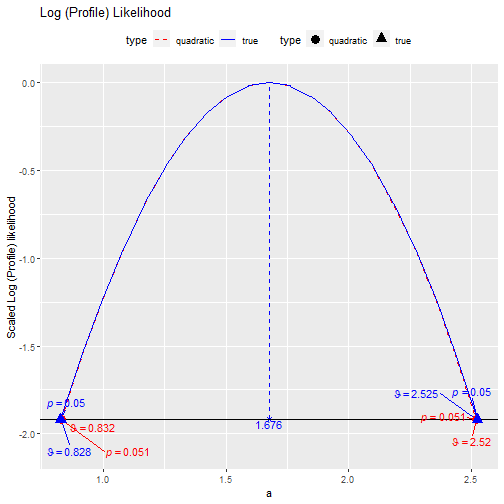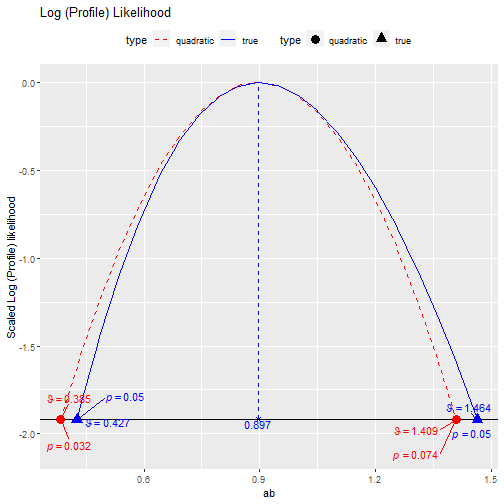# 1 Introduction

This vignette illustrates how to use loglike_compare() in the package semlbci to compare the log profile likelihood of a parameter in a structural equation model (SEM) when it is fixed to a range of values to the log profile likelihood approximated by a quadratic function, using the method by Pawitan (2013).

# 2 Limitations

The function loglike_compare() and its helper functions are for creating illustrative examples and learning only, not for research use. Therefore, they are not as versatile as semlbci() in the types of models and parameters supported. They can only be used for free parameters and user-defined parameters not involved in any constraints. Only a model fitted by maximum likelihood is supported. Last, they will not check whether the computation is appropriate for a model. It is the responsibility of the users to ensure that the computation is appropriate for the model and parameter.

Despite these limitations, they are still useful for generating graphs for illustration and learning.

# 3 Fitting a Simple Mediation model

Suppose a simple mediation model is fitted to the dataset simple_med, came with the package semlbci:

library(lavaan)
library(semlbci)
dat <- simple_med
mod <-
"
m ~ a * x
y ~ b * m
ab := a * b
"
fit <- lavaan::sem(model = mod,
data = simple_med,
fixed.x = FALSE)
parameterEstimates(fit)
#>   lhs op rhs label    est    se      z pvalue ci.lower ci.upper
#> 1   m  ~   x     a  1.676 0.431  3.891  0.000    0.832    2.520
#> 2   y  ~   m     b  0.535 0.073  7.300  0.000    0.391    0.679
#> 3   m ~~   m       34.710 3.471 10.000  0.000   27.907   41.513
#> 4   y ~~   y       40.119 4.012 10.000  0.000   32.256   47.982
#> 5   x ~~   x        0.935 0.094 10.000  0.000    0.752    1.118
#> 6  ab := a*b    ab  0.897 0.261  3.434  0.001    0.385    1.409

# 4 Log Profile likelihood Plots

## 4.1 The a-path

To generate the plot for the a-path coefficient, we can simply call loglike_compare() and setting the parameter to "a".

ll_a <- loglike_compare(fit,
par_i = "m ~ x")

Although teh a-path is labelled, par_i requires the parameter specified in lavaan::model.syntax. Please refer to the help page of loglike_compare.

By default, 21 values will be used to generate the plot. This can be controlled by the argument n_points.

Once the run finishes, we can use plot() to plot the likelihood values:

plot(ll_a, add_pvalues = TRUE)a-path

As expected, the log profile likelihood of the a-path is well-approximated by the quadratic approximation.

## 4.2 The indirect effect

Let us examine the log profile likelihood of the indirect effect. To specify it, we need to use ab :=:

ll_ab <- loglike_compare(fit,
par_i = "ab := ")

Note that it usually take longer for parameter which is a nonlinear function of other parameters, such as an indirect effect, which is the product of two other parameters.

This is the plot:

plot(ll_ab, add_pvalues = TRUE)Indirect Effect

We can see that, as expected, the quadratic approximation is less satisfactory for the indirect effect.

Please refer to the help page of loglike_compare() and its plot method (plot.loglike_compare()), for other available options.

# 5 Final Remarks

The other functions, loglike_range(), loglike_point(), loglike_quad_range(), and loglike_quad_point() are helper functions used by loglike_compare(). They are exported such that interested users can use them to compute the points directly.

More examples can be found in the “examples” folders in the OSF page for this package and Cheung and Pesigan (2023). Some of the figures in the examples were generated by these functions.

# 6 Reference

Cheung, S. F., & Pesigan, I. J. A. (2023). semlbci: An R package for forming likelihood-based confidence intervals for parameter estimates, correlations, indirect effects, and other derived parameters. Structural Equation Modeling: A Multidisciplinary Journal. Advance online publication. https://doi.org/10.1080/10705511.2023.2183860

Pawitan, Y. (2013). In all likelihood: Statistical modelling and inference using likelihood. Oxford University Press.